﻿

### Linear natural liftings of forms to Weil bundles with Weil algebras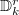${\mathbb D}^r_k$

#### Abstract

We give an explicit description and calculate the  dimension of the vector space of linear natural liftings of$p$-forms on$n$-dimensional manifolds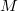$M$ to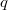$q$-forms on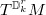$T^{{\mathbb D}^r_k}M$, where${\mathbb D}^r_k$ is the Weil algebra of$r$-jets at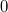$0$ of smooth functions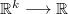${\mathbb R}^k\longrightarrow{\mathbb R}$,  for all non-negative integers$n$,$p$,$q$,$r$,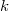$k$ except the case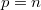$p=n$  and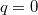$q=0$.

DOI Code: 10.1285/i15900932v28n2p99

Keywords: natural operator; Weil algebra

Classification: 58A32

Full Text: PDF